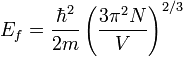# Fermion Gas well potential

## Homework Statement

For free electrons in a metal, the depth of a potential well can be determined by observing that the work function is the energy required to remove an electron at the top of the occupied states from the metal; an electron in this state has the Fermi energy.

Assuming each atom provides one free electron to the gas, find the depth of the well for a free electron in gold (work function = 4.8 eV)

## Homework Equations## The Attempt at a Solution

I'm assuming that the answer will be just the fermi energy plus the work function, but I can't seem to get the right answer when plugging everything in.

For N/V, I used (Avogadro # x Density of gold) / (molar mass of gold) , and got 3.89 x 10^9 electrons / cubic meter

The answer should be on the order of 1 ev, but I can't seem to get this. The m here refers to the mass of a single electron, correct? But using that, I just don't get the right orders of magnitude. (I get about 5 x 10^6).

That isn't nearly enough electrons in a cubic meter. You made a mistake in calculating N/V. Please show all of your work so we can see what you did wrong.

Yeah you're right. 10^9 was what I got when I took number of electrons / m^3 and raised it to the 1/3 power, to get N/L. I think I'm supposed to use the 1 dimensional equation.

edit: yeah I was just being dumb, I had it right, was just using the wrong units for mass. Thanks though.

Last edited:
You have the right equation. Just show your work on solving N/V so we can check it. Remember to show all units.